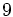# General linear group:GL(2,Z9)

View a complete list of particular groups (this is a very huge list!)[SHOW MORE]

## Definition

This group can be defined in the following equivalent ways:

1. It is the group$GL(2,\mathbb{Z}_9)$ or$GL(2,\mathbb{Z}/9\mathbb{Z})$, i.e., the general linear group of degree two over the ring of integers modulo$9$.
2. It is the group$GL(2,\mathbb{F}_3[t]/(t^2))$, i.e., the general linear group of degree two over the ring$\mathbb{F}_3[t]/(t^2)$.

Note that although the rings in question are different, the corresponding general linear groups are isomorphic.

## Arithmetic functions

Want to compare and contrast arithmetic function values with other groups of the same order? Check out groups of order 3888#Arithmetic functions

### Basic arithmetic functions

Function Value Similar groups Explanation
order (number of elements, equivalently, cardinality or size of underlying set) 3888 groups with same order As$GL(2,R)$,$R$ a discrete valuation ring of length$l = 2$ with residue field of size$q = 3$:$q^{4l - 3}(q - 1)(q^2 - 1) = 3^5(2)(8) = 3888$
exponent of a group 72 groups with same order and exponent of a group | groups with same exponent of a group
nilpotency class -- not a nilpotent group
derived length 4 groups with same order and derived length | groups with same derived length

## GAP implementation

### Other descriptions

Description Functions used
GL(2,ZmodnZ(9)) GL and ZmodnZ